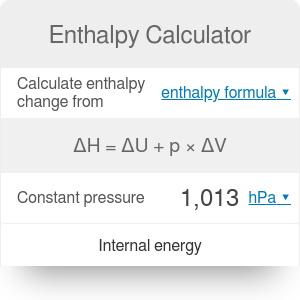Change in internal energy
J
Change in volume
US gal
Pressure
bar
Change in enthalpy
J

# Enthalpy Calculator

By Bogna Haponiuk

This enthalpy calculator will help you calculate the change in enthalpy of a reaction. Read on to learn how to calculate enthalpy and what is its definition. We will also explain the difference between endothermic and exothermic reaction, as well as provide you with an example of calculations.

Check out our ideal gas law calculator, too!

## What is enthalpy?

Enthalpy measures the total energy of a thermodynamic system - either in the form of heat or volume multiplied by pressure. It is a state function, depending only on the equilibrium state of a system.

The more interesting quantity is the change of enthalpy - the total energy that was exchanged within a system. It is a simplified description of the energy transfer (energy is in the form of heat or work done during expansion).

## Endothermic or exothermic reaction?

There are two main types of thermodynamic reactions: endothermic and exothermic. An endothermic reaction causes absorption of heat from the surroundings. An exothermic one releases heat to the surroundings.

Both these reaction types cause energy level differences and therefore differences in enthalpy. All you need to remember for the purpose of this calculator is:

• If the reaction is endothermic, change in enthalpy is positive, as heat is gained (absorbed from the surroundings).
• If the reaction is exothermic, change in enthalpy is negative, as heat is lost (released to the surroundings).

## Enthalpy formula

Enthalpy, be definition, is the sum of heat absorbed by the system and the work done when expanding:

`H = Q + pV`

where Q stands for internal energy, p for pressure and V for volume.

If you want to calculate the change in enthalpy, though, you need to consider two states - initial and final. We will assume that the pressure is constant while the reaction takes place. Then, the change in enthalpy is actually

`ΔH = (Q₂ - Q₁) + p * (V₂ - V₁)`

or, simplified,

`ΔH = ΔQ + p * ΔV`

where:

• Q₂ and V₂ are the internal energy and volume of the products of the reaction;
• Q₂ and V₂ are the internal energy and volume of the reactants;
• p is the constant pressure;
• ΔQ is the change in internal energy;
• ΔV is the change in volume;
• ΔH is the change in enthalpy.

## How to calculate the enthalpy of a reaction

1. Begin with determining the change of volume of your substance. Let's assume your liquid expanded by 5 liters.
2. Find the change in the internal energy of the substance. Let's say your substance's energy increased by 2000 J.
3. Measure the pressure of the surroundings. We will assume 1 atmosphere.
4. Input all of these values to the equation `ΔH = ΔQ + p * ΔV` to obtain the change in enthalpy:

`ΔH = 2000 J + 1 atm * 5 l = 2000 J + 101,325 Pa * 0.005 m³ = 2506.63 J`

1. You can also open the advanced mode of our enthalpy calculator to find the enthalpy basing on the initial and final internal energy and volume.
Bogna Haponiuk

## Get the widget!

Enthalpy Calculator can be embedded on your website to enrich the content you wrote and make it easier for your visitors to understand your message.

It is free, awesome and will keep people coming back!